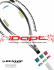# MOVING LOADS - ANALYTICAL AND NUMERICAL APPROACHES!

## Transcription

MOVING LOADS - ANALYTICAL AND NUMERICAL APPROACHES!
```Theoretical Foundations of Civil Engineering - XIV
Ed. by W. Szczesniak, OW PW
Warsaw 2006, pp. 37-48
MOVING LOADS - ANALYTICAL AND NUMERICAL
APPROACHES!
Bartlomiej DYNIEWICZ and Czeslaw BAJER
Institute of Fundamental Technological Research, Polish Academy of Sciences, Warsaw
1
Introduction
and speed of travelling increase. Especially railway and bridge structures are affected by
growing requirements. Dynamic effects in problems with moving load result in increased
deformations. In the case of existing structures the load carrying capacity is a serious
limit for real applications. There are two solutions: rebuild the structure or apply control
system (with active, passive or semi-passive vibration damping). Such solutions have
already been applied in experimental scale and in nature. However, the practical limit in
the development of this technique is in the computational part of the project. Although
limited complexity of the problem (usually reduced to constant travelling speed and simple
set of massless or inertial loads) are intensively investigated, real problems with arbitrary
Analytical solutions of beams and plates under travelling loads are widely presented
in [1, 2, 3]. The reader can find there various problems treated analytically, with broad
list of references. Numerical approach to the problem in the case of standing load can be
found in numerous academic books. However, numerical treatment of the wave problem
in the case of moving force and comparison to analytical solution ir rarely published.
In the paper we intend to compare the accuracy and efficiency of two numerical approaches: discrete mesh method (for example the finite element method) and the meshless
method (moving Galerkin least square method) . Both approaches are related to the
analytical solution. The basic set of problems to be investigated concerns moving massless
and inertial force placed on the string and beam, without and with elastic foundation. In
the present paper we limit our analysis to the string subjected to moving massless force
and inertial force, without or with the Winkler foundation.
lSupported
by 4T12B 04829 grant
-38-
2
Constant moving force (analytical solution)
pr~
The point force moving with a constant
speed c (Fig. 1) is introduced as the Dirac
function o(x). The motion equation then has
the following form:
c
N
N
ill
]
u(x,t)I
L
+
N a 2U(X, t)
-
ax2
A a 2U(X, t) = o(X-d)
at2
p
P
(1)
Figure 1: Constant moving force.
N is the tensile force and pA is the mass density per length. We impose boundary condi-
tions
u(O, t)
and initial conditions
u(x,O)
°
u(l, t)
au(x,t)I
at
°
°
(2)
= 0.
(3)
=
t=O
Fourier transformation
The equation was solved with the use of operational calculus [5J. Antisymmetric part
of the equation (1) was considered. It was written with sinus Fourier transform in the
frequency domain. According to the sinus Fourier transform [6Jwe can get the transform
of the equation (1):
V(j, t)
where:
+ wJ
V(j, t)
j'rrc
W
=
= _!__
pA
sin wt
(4)
(5)
W~
I
J
The Laplace-Carson transformation
In the equation (4) we go to the complex domain. In this case the solution is more simple.
The L-C transformation [7Jallows us to obtain
V.(j
,p
) _ Pw
p
- pA p2 +
1
w2 p2 + wJ .
(6)
Inversion of Laplace-Carson transform
The inversion of L-C transform allows us to return to the real space (6):
V(j),t
P 2 1 2
= -A
p Wj - W
w.)
( smwt
.
- -
Wj
smWjt
(7)
.
The inversion of Fourier transform reduces the equation (7) to time domain:
u(x,t )
2P
= ~L...." -AI
~lP
~
1·
(.
t
W.
~_ 2 smw - --:- smWjt
W
~
).
j'rrx
sm- -·
l
We have the displacement u(x, t) as the final solution of the equation.
(8)
-393
Constant moving load with Winkler foundation (analytical solution)
The differential equation of the string with the Winkler foundation with constant stiffness
k has a form:
2
8 u(x, t)
8t2
82U(X, t)
- N
8x2
+J.L
+
)p
k ( ) _ r(
ux,t
-ox-a.
(9)
The solution of (9) is similar to the solution of the equation (1), with the following
difference:
(10)
J.L
4
Displacement of the string under the moving inertial load (Fig. 2) can be written in the
form:
u(x, t) =
l'
(11)
N
E
where CCx, s) is the Green function. Inertial
load pes, t) applied to the string is given by
the function (12):
p(s,t)
~c
CCx, s) pes, t) ds,
= o(x - a) ( P - m
82u(a
8t2'
t»)
L
u(x,t)I
-j
N
~
L
(12)
4.1
Massless string
Assuming p = 0, P = 1 and x = s, the equation (1) after integration has a form:
u(x) =
-1
N
(H(x - s) x - H(x - s) s)
+ C1 X + C2
,
(13)
where H(x - s) is the Heaviside function. Taking into account boundary conditions (2)
we can solve for constants C1 and C2.
We substitute:
x =a
Ul(t) = u(a, t)
(14)
Taking (11) and (12) with the constant force P - m
the computation of the integral I':
r =
11
C(x,s)o(s
82':1t~t.t),
the problem is reduced to
- a)ds
(15)
Taking into account (14) we have:
C(a,a)
(16)
-40-
and with (11), (12), (14) and (16) we have:
(17)
Static displacement in the middle of the string length:
Pl
(18)
= 4N'
Uo
By introducing (17) and (18) we have dimensionless displacements y(r), and dimensionless
time -r:
ct
y(r) = UI(t)
(19)
r =
z'
Uo
Substitution of (19) into (17) results in:
r(1 - r)ii(r)
+
2ay(r)
where:
4.1.1
8ar(1
- r) ,
(20)
Nl
2mc2'
(21)
= r(1 - r)v(r)
(22)
The case of a =I- 1
We assume the equation
y(r)
to be the solution of (20). Substitution of the function (22) and its second derivative into
(20) we have:
r(1 - r)ii(r)
+
(2 - 4r)v(r)
-
2(1 - a)v(r)
= 8a.
(23)
The Eqn. (23) is the hypergeometric non-homogeneous one [8J. Its general form is given
by the formula:
+ [c -
r(1 - r)ii(r)
(a
+b+
l)rJv(r)
-
=
abv(r)
0 .
(24)
In the first stage we solve a homogeneous equation of the Eqn. (23). With the use of (24)
we obtain the system of equations, which has the following solution:
3 ± yll
2
+ 8a
b
_
1,2 -
3 =t= yll
2
+ 8a
c
=
2 .
(25)
The solution of the hypergeometric equation with c be a natural number c = 1 + m and
a =I- m, b =I- m:
vI(r) = P(a,b,c,r)
pea,
b, c, r) In r
+
f {(a
k)
k=1
+
(1 -
r~ +
(bk) [h(k) _ h(O)J
(Ck)
k.
a) ~1 - b) r} ,
(26)
-41-
where F(a, b, c, r) is the hypergeometric series determined
88:
(27)
(ak)
(bk)
h(k)
= a (a
+ 1)
(a + k - 1)
(b + k - 1)
= b(b + 1)
= 'IjJ(a + k) + 'IjJ(b + k) - 'IjJ(2 + k) - 'IjJ(1 + k)
1
'IjJ(x) = -C
-
+
X
(29)
1
L n (x + n).
00
x
(28)
(30)
n=I
It means that 0: exists for which the solution (26) is not fulfilled.
Particular solution of (23) is as follows:
40:
v.( r)
0: -
(31)
1
The complete solution of the equation (20) with the use of (22) is the equation:
(32)
For the initial condition we can compute constants Al and A2
-40:
A1=--
(33)
1
0: -
The constant A2 = 0 results in the simplification of the formula (32). Finally the displacement of the string with 0: '" 1 is described by the relation
40:
y(r)
0:
-1 r(l
40:
0: _
- r)[l
- vI(r)]
1 r (1 - r) [1 - F(a, b, c, r)]
(34)
where F(a, b, c, r) is given by (27).
4.1.2
The case of
In the case of
0:
0:
=1
= 1 the equation (20) has a form:
r(l
- r)y(r)
+ 2y(r)
=
8r(1 - r)
(35)
The first order equation below is one of two solutions of the Eqn. (35)
r (1 - r)
1Mr)
-
(1 - 2 r) YI ( r ) = O.
(36)
After integration
YI(r)
= r (1 - r)
(37)
The second solution of (35):
YI(r)u(r)
r (1 - r) u(r)
(38)
-42-
After differentiation and substitution of (38) into (35) we have:
+
7(1 - 7)U(7)
= 8
2(1 - 27)U(7)
=
We solve the homogeneous equation (39) substituting Ul(7)
7(1 - 7)Ul(7) + 2(1 - 27)Ul(7)
Ul(7)
=
[7 (1 - 7)t2
7
-
(39)
U(7):
= 0
(40)
(41)
Finally the solution of (39) has a form:
=
U(7)
1
1_
Then
Y2(7) = 27 -
1
21nl-7
7
(42)
7
11 - 27(1 - 7) In--.
7
(43)
7
Since the Wronski determinant W =I- 0, the equations Yl(7) and Y2(7), which are the
integrals of the homogeneous equation are linearly dependent, the general solution of the
equation (35) has a form
(44)
Full solution of Eqn. (35) can be obtained with the use of variation of constant method,
where
(45)
With (37) and (43) we get:
Al(7)
= -
2
2
1-7
8 { 7 - 7 In --
+ In(1 - 7) +
7
!
+ ~ [73 In 1 - 7 _
(7 + 2) 7 - In(l - 7)]} + D,
3
7
2
A2
= ~3
2
7
(3 - 27)
+
D2
(46)
Integration constants Dl and D2 can be computed from initial conditions (3) :
(47)
The final relation for displacement, which is the solution of Eqn. (35) has a form:
4 2
Y(7) = '37 (3 - 27)[27 _ 87(1 - 7) {72
1- 7
1 - 27(1 - 7) In- -1
7
-
-
72 In 1 - 7 + In(1 - 7) +
7
23
+'3[7
1-7
In- 7
1
- '2(7+2)7-ln(I-7)j}
(48)
-43-
5
Meshless methods (Element Free Galerkin Method)
The idea of meshless methods is to eliminate the mesh generation stage, which is the
main disadvantage of the finite element method (or other classical discrete methods). In
the meshless method the set of separated points is placed in the domain of the structure.
Interpolation functions (shape functions) are then generated not in element subdomains,
but in arbitrary placed nodal points.
Below we consider a particular method of shape function generation, called Moving
Least Square (MLS) method . Shape functions are stretched on points being in the
neighbourhood of the given point, in the stepping sub domain O. The interpolation error
node
seta
Figure 3: The set 0 moving along a string.
in MLS method is given by
n
J=
L W(x-x;)
[uh(x,x;)
-
U;]2
,
(49)
i=1
where W(X-Xi) is the weight function, uh(x, Xi) is the polynomial of approximation, and
u; are nodal values. We assume exponential shape functions:
W(x _ Xi)
= {e-(7)2 if (x - x;) :5 1 .
o
if (x - Xi) > 1
(50)
The coefficient a depends on the size of the domain 0 and the numbet of points in the
domain. In the uni-dimensional case
(51)
where monomials in the interpolation polynomial is
pT = (l,x,x2,
... ) ,
(52)
with approximation coefficients
(53)
We minimize the interpolation error. J is minimized according to coefficients a:
8J
8a
= 0
.
(54)
-44Differentiation of (49) yields:
(55)
where:
pTW(X - x.) p
p
=
Pl (Xl)
Pl(.Xl)
A2
=
pTW(X - x.)
P2(X2)
P2(X2)
pm(Xn))
Pm (Xn)
P2(Xn)
Pm (Xn)
.
.
(56)
(57)
(
Pl(Xn)
Equations (51) and (55) lead to:
n
E ¢:(X)
u. .
(58)
.=1
where ¢~(x) is the shape function and k is the degree of the approximation polynomial.
(59)
Since the inversion of the matrix A in a general form can not be simply computed, the
approximation of the zero degree of pT = 1 is applied in practice. Thus we obtain the
so-coiled Shepard function:
¢? = nW(x - x.) .
(60)
2::.=1 W(x - X.)
Eqn. (60) and the differential equation of motion
CT.;,;
+
b, = pil;
(61)
we can obtain the stiffness and inertia matrix:
k;;
'T"T4;
=
=
1
1
Bi N B; dn
¢f pA ¢; dn ,
(62)
where B = ~, N - tensile force and p - mass density. Nodal matrices k and m computed
for domains n are assembled in global matrices K and M. The proper choice of (.¥
parameter is still the fundamental problem (Eqn. 50).
6
Results
Numerical results are compared with analytical results. In the Fig. 4 we have plots of
displacemens under moving force and in the middle of the string length. The analytical
solution well coincides with the one obtained with the finite element method or central
difference method. The meshless method results in good approach of the accurate solution.
In this method we can notice the phace error, visible especially in the second half of the
observation period. It means that the rigidity is higher than required. However, smoothing
effect is the main reason of differences of results. Significantly lower number of points in
-45(1)
I
-0.2
,
-0.4
,,"
-O.S
" ..""
-0..
,,--
(2)
analyt!cally -
~~~\~~I ~·--
,
········_··t
''''--,,·'i'·"''''''''''"''''-"i"·-''-""-"-t
""''''"''''"1''"
t-""-,,I,,·-,,-·,,+
-1.2
·''''
"
_-_
-0.5
----1
~"""-."""""".c+:,,,·--------··,...,··---------t·········------
-1.4
_--t-I .
--.- ..-..-----.
__.._-_..
-1.8
·1.•
.."
I....""" ..__ """.l"._ ..".." ..".."j ",, .
i
!
!
i
"·"..·["·,,"",,..,,"--·t ....·,,---""+"-·--"""-"-]-,,·
" .-""-,,
""_ .....
.1
-1.5
r"""""--"-'+""-"-'"-'''''
V1IL
Figure 4: Displacements under constant moving force: (1) under moving force, (2) in the
middle of the string length (number of points in n equal to 10).
(1)
12)
0.2
-0.2
-0.4
-O.B
-0••
-0.2
-0.4
-0.6
·0 .•
·1
-1.2
-1.2
-1.4
-1.4
·1.6
-1.6
-1.8
·1 .a
Figure 5: Displacements under constant moving force: (1) under moving force, (2) in the
middle of the string length (number of points in n equal to 2).
the moving domain (2 instead of 10 as in the previous case) (Fig. 5) results in quite
similar plots. Both the amplitude and the period of vibrations are acceptable.
The numerical approach in the case of Winkler foundation does not differ from the
analytical solution. We must emphasize here, that continuously moving force in the
numerical application is replaced with the sequence of marching pair of forces, applied
to nodal points. In such a case we neglect mixed derivatives of the formulation. We can
notice that such an analysis does not introduce significant error (Fig. 6).
Plots for the inertial load in the case of moving high mass (Fig. 7) differ with analytical
ones. In the case of lower moving mass both lines coincede (Fig. 8). Higher speed does
not allow to compare both analytical and numerical plots successfully (Fig. 8).
7
Conclusions
Analytical solutions fail in cases of high speed orhigh inertia of the moving force. Meshless
methods are fast and can be successfully applied to the group of problems discussed in
the paper.
-46-
III
121
0.05
-O.OS
-0.05
-0.1
-D.l
-D.1S
-D.1S
-0.2
-D.2
-D.25
-D.25
-0.3
-D.3
-0.36
-D.36
-0.'
0
0.1
0.6
0.2
0.7
0.6
I
0.9
'l1li.
Figure 6: Displacements under constant moving force - string based on the Winkler
foundation: (1) under moving force, (2) in the middle of the string length.
1"
,_.
i
.1._..
121
numortca~~~
•__ J.....
I
!
-
._~--__
-0.2
-0.'
---------
..
+-.----------+ -" ..--..:~",~.,==~
-.---_I
i
1
"j·····--------·t··---------:" .. ol······ .. --- ..--+-....··....--..·..
-0.6
-0.&
····.. ----·--
..
r..·····
.: ::==::::-r·:::::::::l·:·:::::::::l··::::::::::C:::::·-- .
•2.5
L-_--''--_--'- __
o
0.2
0.4
-'-__
0.6
--'__
0.8
.....J
-1.4
o
D.'
0.2
0.8
0.&
Wl
131
vtlL
Figure 7: Inertial force (string mass/moving mass=1/1000) , 0:
2) c = 0.1; 3) c = 0.04.
=1=
1; the speed: 1) c = 0.01;
-47-
(2)
(1)
.(l.2
.(l.4
-o.s
-o.•
-1
-1.2
-1.6
-1.•
0.2
0.4
O.B
D.•
YIIL
Figure 8: Inertial load with string mass/moving mass=I/10
of the force. Left diagram: v = 0.1, right diagram: v = 0.5.
(a =I- 1) for different speed
Figure 9: Inertial load, high moving speed (a = 1)
-48-
References
[1J L. Fryba.
Prague,
1972.
[2J W. Szczesniak.
Warszawska,
Inercyjne obcizenia ruchome
budownictwo 112, 1990.
na belkach,
Prace Naukowe,
Politechnika
[3J W. Szczesniak.
Wybrane
plyt poddanyeh
inercyjnym
obcizeniom ruchomym, Prace Naukowe, Politeehnika Warszawska, budownictwo 119,
1992.
[4J T. Belytschko,
Y. Krongauz, D. Organ, M. Fleming, and P. Krysl. Meshless methods:
An overview and recent developments.
Comput. Methods Appl. Mech. Engrg, 139(14):3-47, July 1996.
[5J K. W. Wagner.
1960.
[6J R. Bracewell.
Przeksztalcenie Fouriera i jego zastosowania. WNT, Warszawa,
[7J G. Doetsch.
[8J
Rachunek operatorowy i przeksztalcenie Laplace'a. PWN, Warszawa,
Praktyka przeksztalcenia Laplace'a. PWN, Warszawa,
1964.
Hypergeometric functions and their applications. Springer,
J.B. Seaborn.
1965.
New York,
1991.
RUCHOME
OBCIJ\ZENIA
- ROZWINIECIA
ANALITYCZNE
I NUMERYCZNE
W pracy przedstawiono
ocene dokladnosci i skutecznosci zastosowania metod numeryczfalowych. Metody analityczne sa, skuteczne w ograniczonym
zakresie
parametr6w.
Klasyczne metody numeryczne pozwalaja uzyskaC wysoka dokadnosc, choe
wymagaja znacznie wiekszego wysilku obliczeniowego niz metody bezsiatkowe.
Te ostatnie
bledem fazowym oraz charakteryzuja
sie rozmyciem czola fali.
```

### Flemish String Board Attachment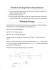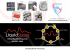### Knotted Friendship Bracelet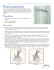### the ultimate guitarist`s upgrade manual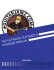### Double Bass Survival Guide By David Gage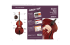### See Full PDF - The Clean Vape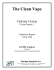### think forward Sample Tracking and Information### Outbound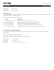### March 1995 - Utah Racquetball### here - Concurrent Dynamics### I/O and serialization Streams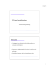### Application of Inertial Forces for Generating Unidirectional Motion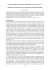### The Analytical Scientist### Bridge Tailpiece Vintage Vibrato Bridge Bridge Location### Chapter 2 Abstract Data Types### Guitar Method - Learning Guitar Now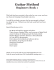### Stress concentration factors for pin lever of runner blade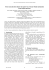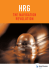### Secure Coding Practices for Middleware### ownyourgame - Dunlop Portal